Ch. 1 - A Review of General ChemistryWorksheetSee all chapters
 Ch. 1 - A Review of General Chemistry 4hrs & 47mins 0% complete Worksheet Ch. 2 - Molecular Representations 1hr & 12mins 0% complete Worksheet Ch. 3 - Acids and Bases 2hrs & 45mins 0% complete Worksheet Ch. 4 - Alkanes and Cycloalkanes 4hrs & 18mins 0% complete Worksheet Ch. 5 - Chirality 3hrs & 33mins 0% complete Worksheet Ch. 6 - Thermodynamics and Kinetics 1hr & 19mins 0% complete Worksheet Ch. 7 - Substitution Reactions 1hr & 46mins 0% complete Worksheet Ch. 8 - Elimination Reactions 2hrs & 24mins 0% complete Worksheet Ch. 9 - Alkenes and Alkynes 2hrs & 10mins 0% complete Worksheet Ch. 10 - Addition Reactions 3hrs & 33mins 0% complete Worksheet Ch. 11 - Radical Reactions 1hr & 57mins 0% complete Worksheet Ch. 12 - Alcohols, Ethers, Epoxides and Thiols 2hrs & 34mins 0% complete Worksheet Ch. 13 - Alcohols and Carbonyl Compounds 2hrs & 14mins 0% complete Worksheet Ch. 14 - Synthetic Techniques 1hr & 28mins 0% complete Worksheet Ch. 15 - Analytical Techniques: IR, NMR, Mass Spect 7hrs & 18mins 0% complete Worksheet Ch. 16 - Conjugated Systems 5hrs & 49mins 0% complete Worksheet Ch. 17 - Aromaticity 2hrs & 24mins 0% complete Worksheet Ch. 18 - Reactions of Aromatics: EAS and Beyond 4hrs & 31mins 0% complete Worksheet Ch. 19 - Aldehydes and Ketones: Nucleophilic Addition 4hrs & 54mins 0% complete Worksheet Ch. 20 - Carboxylic Acid Derivatives: NAS 2hrs & 3mins 0% complete Worksheet Ch. 21 - Enolate Chemistry: Reactions at the Alpha-Carbon 1hr & 59mins 0% complete Worksheet Ch. 22 - Condensation Chemistry 2hrs & 13mins 0% complete Worksheet Ch. 23 - Amines 1hr & 43mins 0% complete Worksheet Ch. 24 - Carbohydrates 5hrs & 56mins 0% complete Worksheet Ch. 25 - Phenols 15mins 0% complete Worksheet Ch. 26 - Amino Acids, Peptides, and Proteins 2hrs & 54mins 0% complete Worksheet

# Formal Charges

See all sections

We use our knowledge of valance electrons to determine what he formal charge of the molecule will be.

###### Formal and Net Charge

Concept #1: Calculating formal and net charge.

Transcript

So now that we understand bonding preferences so well, I want to move toward a really related topic called formal charges. Formal charges are just based on the entire idea of bonding preferences, so let's go ahead and just jump right into it.
Basically, a formal charge is a sign whenever there's a difference between the number of valence electrons an atom wants to have and the number of valence electrons it actually has. So remember that the group number is how many it wants, the valence electrons, the sticks, and the dots, are the amount it actually has.
All you do to calculate formal charge is you take the group number whatever that is, that could be group 4, group 5, whatever and then you subtract the valence electrons which is just the sticks and the dots. It's really simple math. A lot of times we'll just be able to do this on your fingers, actually all the time.
You take your group number and then you just subtract the sticks and the dots and you're good. Alright. That's called the formal charge.
The net charge, let's write that down, the net charge is the term that we give for the sum of all the formal charges. Now this is an important point because I remember when I was an undergrad, I used to get a little bit confused over like, “Oh, does the formal charge go on the whole thing or is it just one atom?” No, the formal charge is one atom at a time. You're just looking at each atom and saying does this have a formal charge, does this have a formal charge. The net charge is the collection of all of those summed together.
Okay? Hopefully not too complicated. There's usually not that many formal charges on a molecule.

Easiest formal charge formula you will find anywhere on the internet:The Net Charge is the sum of all the formal charges on a molecule.

Calculate the formal charge of the following molecule: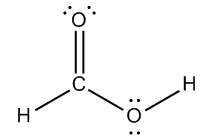Concept #2: Calculate the formal charges of ALL atoms.

Transcript

Let's go ahead and do this example where I want to look at each atom and I want to count the formal charges for all of them. What that means is I'm going to be looking at group numbers and I'm going to be looking at sticks and dots.
Let's start off with the hydrogens. Even though we already know that this fits the bonding preference. Remember that bonding preferences say that hydrogen wants to have what? One stick, one bond. But let's just do it anyway.
Hydrogen is in what group? One. How many sticks does it have? One. How many dots does it have? Zero. So it's going to be one, according to my equation. My equation says that formal charge equals group number which is one, minus sticks and dots, which is one, so that's zero. So this has a zero formal charge. Do you see that? But it also fits with my bonding preference. As long as your bonding preference agrees with what you see, that's going to be zero.
Let's do this with this oxygen right here. First of all, does this oxygen fit my bonding preference according to bonding preferences? Yes, it does. It already fits it so I can already put a zero here. But now I want to show you mathematically how it works out too.
We said that the formal charge equals the group number. What's the group number of oxygen? It's 6. Minus the number of sticks and dots. So how many is that altogether? That's 6. So then I would get a formal charge of zero.
See how easy that is. So now you do that for carbon. But is carbon fulfilling its bonding preference? It already is, so I can just put a zero there.
Then finally, there was a typo, just so you know. So notice that in your page, the oxygen did not have those lone pairs, go ahead and just write those in. That is just didn't copy. Okay?
Basically for this one, the oxygen has 6 electrons total. It wants 6 electrons, I'm sorry, and it has 6 electrons total. In terms of the valence, sticks and dots, so this would also be zero. This is another way that you can fulfill your bonding preference by having a double bond and having two lone pairs. It still counts as two bonds and two lone pairs.
So I hope that you guys see how if it fits its bonding preference, you don't need to calculate, but calculating is always just safe to do.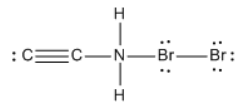Practice: Calculate the net charge of the molecule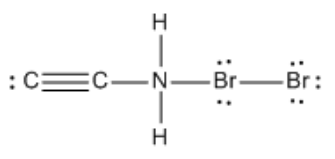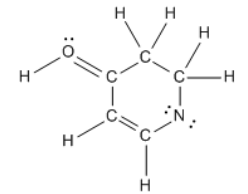Practice: Calculate the total charge of the molecule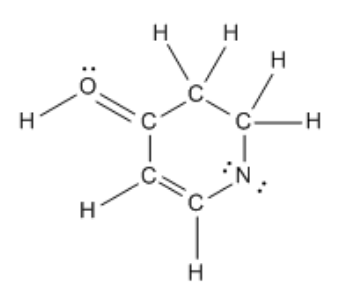Hell yeah! That’s literally all there is to know. Don’t let any professors complicate it more for you.